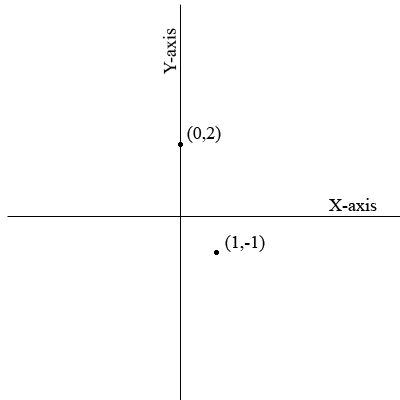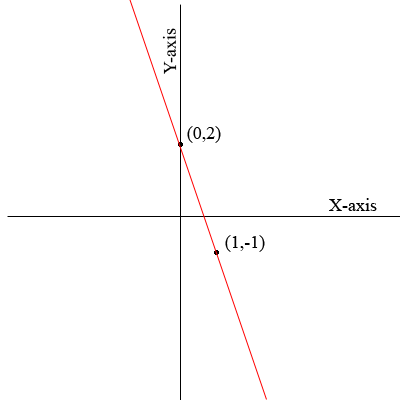SEARCH HOMEMath Central Quandaries & QueriesQuestion from Penehafo : I have a problem of drawing a straight line graph by the equation y=2x-1Hi Penehafo,

Since you know that the graph is a straight line you need only plot two points on the line and then join them using a ruler. I am going to illustrate using a different equation $y = -3x + 2.$ I can use whatever two points on the graph that I choose so I am going to choose points that make the arithmetic easy.

When $x = 0$ we have $y = -3 \times 0 + 2 = 2$ and hence the point $(0,2)$ is on the graph.

When $x = 1$ we have $y = -3 \times 1 + 2 = -1$ and hence the point $(1,-1)$ is on the graph.

Plotting these points givesand connecting the points I getNow try your equation,
PennyMath Central is supported by the University of Regina and The Pacific Institute for the Mathematical Sciences.